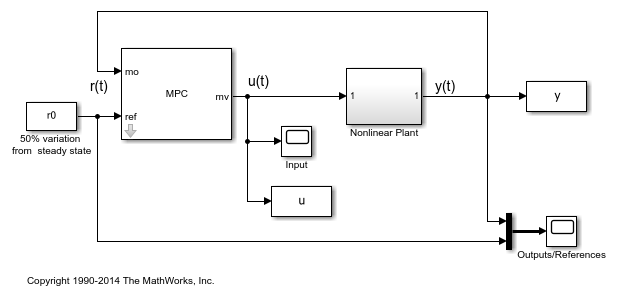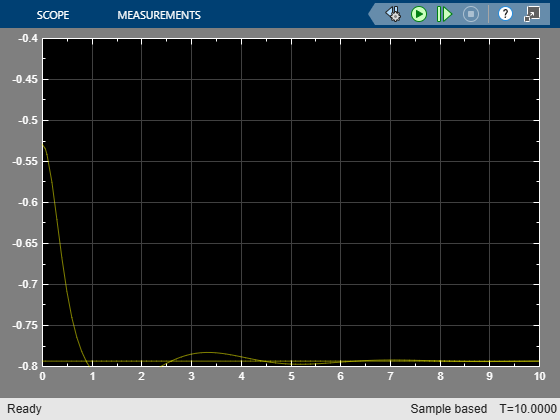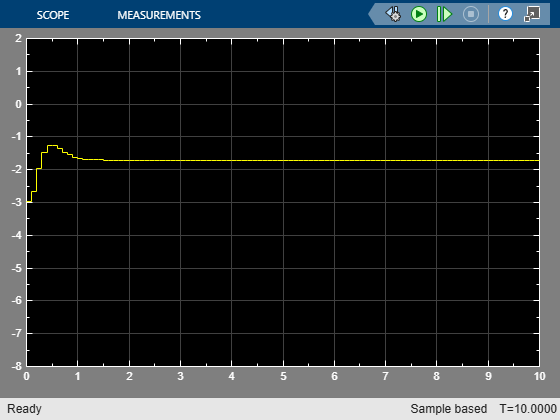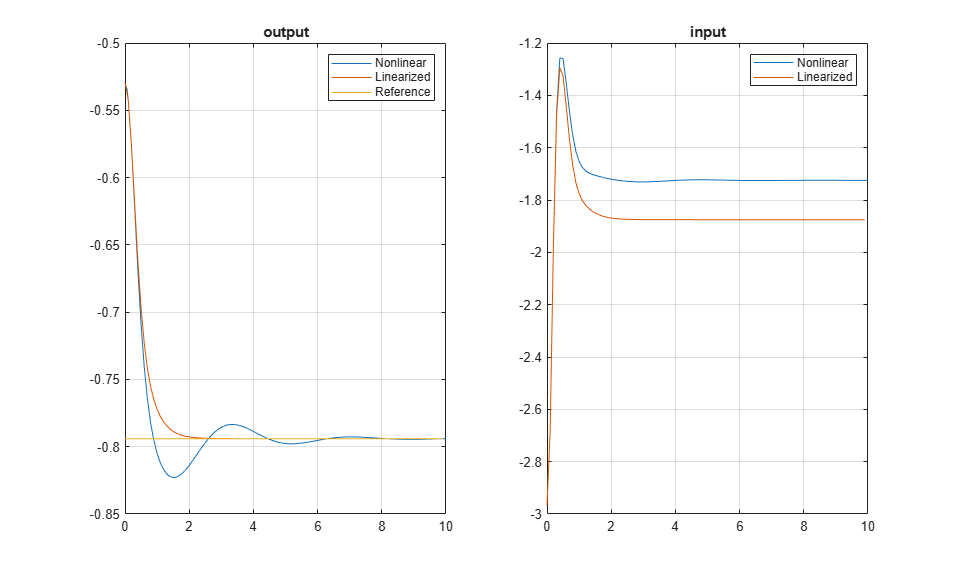Design Model Predictive Controller at Equilibrium Operating Point

This example shows how to design a model predictive controller with nonzero nominal values.

The plant model is obtained by linearization of a nonlinear plant in Simulink® at a nonzero steady-state operating point.

Linearize Nonlinear Plant Model

disp('Simulink is required to run this example.')
return
end
if ~mpcchecktoolboxinstalled('slcontrol')
disp('Simulink Control Design is required to run this example.')
return
end

The nonlinear plant is implemented in Simulink model mpc_nloffsets and linearized at the default operating condition using the linearize function from Simulink Control Design.

Create operating point specification for the current model initial condition.

plant_mdl = 'mpc_nloffsets';
op = operspec(plant_mdl);

Compute the operating point for this initial condition.

[op_point, op_report] = findop(plant_mdl,op);
Operating point search report:
---------------------------------

opreport =

Operating point search report for the Model mpc_nloffsets.
(Time-Varying Components Evaluated at time t=0)

Operating point specifications were successfully met.
States:
----------
<strong>Min</strong>        <strong>x</strong>       <strong>Max</strong>    <strong>dxMin</strong>        <strong>dx</strong>         <strong>dxMax</strong>
<strong>____</strong>    <strong>_______</strong>    <strong>___</strong>    <strong>_____</strong>    <strong>___________</strong>    <strong>_____</strong>

(1.) mpc_nloffsets/Integrator
-Inf    0.57514    Inf      0      -1.8208e-14      0
(2.) mpc_nloffsets/Integrator2
-Inf     2.1503    Inf      0      -8.3846e-12      0

Inputs:
----------
<strong>Min</strong>       <strong>u</strong>       <strong>Max</strong>
<strong>____</strong>    <strong>______</strong>    <strong>___</strong>

(1.) mpc_nloffsets/In1
-Inf    -1.252    Inf

Outputs:
----------
<strong>Min</strong>        <strong>y</strong>        <strong>Max</strong>
<strong>____</strong>    <strong>________</strong>    <strong>___</strong>

(1.) mpc_nloffsets/Out1
-Inf    -0.52938    Inf

Extract nominal state, output, and input values from the computed operating point.

x0 = [op_report.States(1).x;op_report.States(2).x];
y0 = op_report.Outputs.y;
u0 = op_report.Inputs.u;

Linearize the plant at the initial condition.

plant = linearize(plant_mdl,op_point);

Design MPC Controller

Create an MPC controller object with a specified sample time Ts, prediction horizon p, and control horizon m.

Ts = 0.1;
p = 20;
m = 3;
mpcobj = mpc(plant,Ts,p,m);
-->The "Weights.ManipulatedVariables" property of "mpc" object is empty. Assuming default 0.00000.
-->The "Weights.ManipulatedVariablesRate" property of "mpc" object is empty. Assuming default 0.10000.
-->The "Weights.OutputVariables" property of "mpc" object is empty. Assuming default 1.00000.

Set the nominal values in the controller.

mpcobj.Model.Nominal = struct('X',x0,'U',u0,'Y',y0);

Set the output measurement noise model (white noise, zero mean, variance = 0.01).

mpcobj.Model.Noise = 0.1;

Set the manipulated variable constraint.

mpcobj.MV.Max = 0.2;

Specify the reference value for the output signal.

r0 = 1.5*y0;

Open and simulate the model.

mdl = 'mpc_offsets';
open_system(mdl)
sim(mdl)
-->Converting model to discrete time.
-->Assuming output disturbance added to measured output channel #1 is integrated white noise.Simulate Using sim Command

Simulate the controller.

Tf = round(10/Ts);
r = r0*ones(Tf,1);
[y1,t1,u1,x1,xmpc1] = sim(mpcobj,Tf,r);

Plot and compare the simulation results.

subplot(1,2,1)
plot(y.time,y.signals.values,t1,y1,t1,r)
legend('Nonlinear','Linearized','Reference')
title('output')
grid
subplot(1,2,2)
plot(u.time,u.signals.values,t1,u1)
legend('Nonlinear','Linearized')
title('input')
gridbdclose(plant_mdl)
bdclose(mdl)